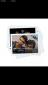# whats the differences between gravitational constant and gravitational acceleration

2 posts / 0 new
Vín Hásnatwhats the differences between gravitational constant and gravitational acceleration

what's the differences between gravitational constant and gravitational acceleration,at leat 10 differences pls..

Jhun Vert• Mathematics inside the configured delimiters is rendered by MathJax. The default math delimiters are $$...$$ and $...$ for displayed mathematics, and $...$ and $...$ for in-line mathematics.